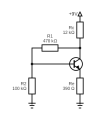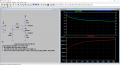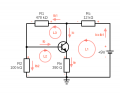# Basic C-feedback circuit question

#### kiltro

Joined Oct 24, 2011
48
So, I was studying BJT collector feedback configuration, but then looking in some schematic I come into this...So in my understanding it's not the standard c-feedback configuration (because there is R2) and it's not the standar DVB configuration (because R1 doesn't go to VCC, right?

My question is, can I use the usual c-feedback equations? Otherwise how would you go about it?

Thanks

#### tindel

Joined Sep 16, 2012
909
You cannot use the standard equations. Sounds like you're studying this... is this for a class? Have you tried to solve this and derive the equations?

•kiltro and dcbingaman

#### tindel

Joined Sep 16, 2012
909
For practice, I did the math on this. Appears to be homework problem of some sort, as the resulting numbers are very round. However, I cheated a little bit to simplify the calculations. I assumed the transistor was ideal (Ib=0 and Vbe=0.7V). A quick LTSpice sim showed that I was within a few mV of the correct solution with both a 2N2222 and a 2N3904.

•kiltro

#### WBahn

Joined Mar 31, 2012
28,166
My question is, can I use the usual c-feedback equations? Otherwise how would you go about it?
Think about this a bit. If you can just throw the usual c-feedback equations at it, then you are saying that the addition of that 100 kΩ resistor has NO impact on the circuit's behavior at all. While it's possible that this particular resistor has such a large value that it's impact is negligible (though I doubt that's the case given the size of the emitter resistor and the likely current-gain of the transistor, as well as the size of the collector-base resistor), what if that resistor were 10 kΩ or even smaller?

There comes a point where you have to not scrounge through your stock of equations and just analyze the circuit.

•kiltro

#### kiltro

Joined Oct 24, 2011
48
Thanks both for helping!

It's not homework in the sense I'm studying for school, it's just for personal intrtest and yes it's an actual circuit (big muff pi).

Is there any good source that explain this?

#### WBahn

Joined Mar 31, 2012
28,166
Thanks both for helping!

It's not homework in the sense I'm studying for school, it's just for personal intrtest and yes it's an actual circuit (big muff pi).

Is there any good source that explain this?
What do your circuit analysis skills look like?

Are you comfortable with the transistors enough to know the different regions of operation and the conditions that apply in in each?

Are you comfortable with Kirchhoff's voltage and current laws?

Have you been exposed to analysis techniques such as node voltage or mesh current analysis?

Are you comfortable doing algebra involving a few equations and a few unknowns?

•kiltro

#### kiltro

Joined Oct 24, 2011
48
What do your circuit analysis skills look like?

Are you comfortable with the transistors enough to know the different regions of operation and the conditions that apply in in each?

Are you comfortable with Kirchhoff's voltage and current laws?

Have you been exposed to analysis techniques such as node voltage or mesh current analysis?

Are you comfortable doing algebra involving a few equations and a few unknowns?
I've studied electronics and telecomunications in high school (I'm now 37) and I've brought the subject back out

1. I've currently started studying two books "Introductory Circuit Analysis, Boylestad R." and "Electronic Principles, Malvino A.P."
I see online that there are more specific books on the subject, mainly old ones (Analysis and Design of Transistor Circuits, Transistor Circuit Analysis and Design, etc...).

2. Yes

3. Yes

4. Yeah, but I might have to review

5. Yes

6. Not so much

#### LvW

Joined Jun 13, 2013
1,621
Is there any good source that explain this?
Because your answer to WBahns questions were - more or less - "yes", you do not need another source for explaining the circuit.
At first, you should try to understand WHY the shown configuration provides negative feedback.
Then - as a next step - you can write down the relevant Kirchhoff equations. Show us the results and - if necessary - we can correct you.
Then, following WBahns procedure you can do some "algebra involving a few equations and a few unknowns".

•kiltro

#### ericgibbs

Joined Jan 29, 2010
17,144
Last edited:
•kiltro

#### kiltro

Joined Oct 24, 2011
48
hi kiltro.
As this is not homework, but personal study, this is what LTSpice shows for that example in the last link.

Ask if you have a query.
E
View attachment 284366
Thank everyone for replies!

Yes I simulated it with LTSpice, but I'd like to train solving by pen.
I'll try putting down equations like @LvW suggested, and use use you link as a reference/check•ericgibbs

#### BobaMosfet

Joined Jul 1, 2009
2,063
So, I was studying BJT collector feedback configuration, but then looking in some schematic I come into this...

View attachment 284347
So in my understanding it's not the standard c-feedback configuration (because there is R2) and it's not the standar DVB configuration (because R1 doesn't go to VCC, right?

My question is, can I use the usual c-feedback equations? Otherwise how would you go about it?

Thanks
Something to remember while you examine schematics- Pay attention to voltage dividers. They are EVERYWHERE in circuits. In your example, Rc+R1 and R2 on the other side of the base form a voltage divider controlling base voltage.

Title: Understanding Basic Electronics, 1st Ed.
Publisher: The American Radio Relay League
ISBN: 0-87259-398-3

•kiltro

#### tindel

Joined Sep 16, 2012
909

Assume an ideal npn transistor (Ib=0 and Vbe=0.7V). And note the following:
Vb ~= 1/6*Vc (voltage divider)

There are then three equations to consider:
Ir1 = Ir2 = (Vc - 1/6*Vc) / 470kohm
Ire = (1/6*Vc - Vbe) / 390ohm
Vc = 9V - (Ir1 + Ire) * 12kohm

Now there are three equations and three unknowns (Vc, Ir1, Ire)... and is basic algebra to do the rest.

My hand calculated results were Vc=4.96V and Ve=125mV

As you can see from my results, I arrived at reasonable solution without even considering the gain of the transistor. Now in real life the transistor does have gain, and additional current will flow into the base as the gain goes down (all things being equal). To derive that, I recommend using the Thevenin equivalent circuit of the R1/R2 voltage divider to keep the math linear with a reasonable number of equations to keep track.

Where it really gets interesting is how the circuit performs over a range of beta's - which is the reason you'd use this circuit in the first place - that is with that additional feedback.
Using the advice above, you should be able to derive something close to the following equation:

Vc ~= 9-4*(beta/(34.8-beta))

This equation shows that with an ideal transistor (beta=infinity), Vc = 5V, and with a minimum beta of 40 (per this datasheet, @25degC, @100uA Ic) Vc ~=7V. That's a relatively stable output voltage over a wide range of beta. This can then be used as an amplifier in a wide range of applications. Generally, an AC signal will be coupled into the base using a coupling capacitor. That's where circuits like this really excel.•kiltro

#### WBahn

Joined Mar 31, 2012
28,166

A place to start for getting a first-cut answer is to assume that there is no base current in the transistor and then to assume that the current that splits off at the collector is small enough so that we can assume that whatever current flows through the 12 kΩ resistor is what flows through the collector-emitter ports of the transistor and on through the emitter resistor.

IF those two assumptions are good enough, then solving the circuit becomes very simple.

BUT, after doing so we need to see if those two assumptions are actually reasonable and, if not, we need to remove whichever ones aren't.

With these assumptions, we have the following in terms of the collector voltage:

I prefer to work symbolically as far as possible because it, (1) gives me the most bang-for-the-buck in terms of units tracking to check my work, (2) let's be see what parts of things are major and minor contributors to the results.

$$V_B \; = \; I_C \cdot R_e \; + \; V_{BE} \\ V_C \; = \; V_{CC} \; - \; I_C \cdot R_c \\ V_B \; = \; V_C \cdot \frac{R_2}{R_1+R_2}$$

From this we can set the first and third equation equal to each other and use the second equation to eliminate Ic from the result.

$$V_C \cdot \frac{R_2}{R_1+R_2} \; = \; I_C \cdot R_e \; + \; V_{BE} \\ I_C \; = \; \frac{V_{CC} \; - \; V_C}{R_c}$$

This leaves us with one equation in one unknown, namely Vc:

$$V_C \cdot \frac{R_2}{R_1+R_2} \; = \; \frac{V_{CC} \; - \; V_C}{R_c} \cdot R_e \; + \; V_{BE}$$

Cranking some algebra:

$$V_C \cdot \frac{R_2}{R_1+R_2} \; = \; V_{CC} \frac{R_e}{R_c} \; - \; V_C \frac{R_e}{R_c} \; + \; V_{BE} \\ V_C \cdot \frac{R_2}{R_1+R_2} \; + \; V_C \frac{R_e}{R_c} \; = \; V_{CC} \frac{R_e}{R_c} \; + \; V_{BE} \\ V_C \left( \frac{R_2}{R_1+R_2} \; + \; \frac{R_e}{R_c} \right) \; = \; V_{CC} \frac{R_e}{R_c} \; + \; V_{BE} \\ V_C \; = \; \frac{V_{CC} \frac{R_e}{R_c} \; + \; V_{BE}}{\left( \frac{R_2}{R_1+R_2} \; + \; \frac{R_e}{R_c} \right)}$$

Now it's time for some numbers:

$$\frac{R_e}{R_c} \; = \; \frac{390 \; \Omega}{12 \; k\Omega} \; = \; 0.0325 \\ \frac{R_2}{R_1+R_2} \; = \; \frac{100 \; k\Omega}{470 \; k\Omega \; + \; 100 \; k\Omega} \; = \; 0.17544$$

From this we can see that the numerator will be dominated by Vbe and the denominator will be dominated by the base-bias voltage divider.

$$V_C \; = \; \frac{9 V \cdot 0.0325 \; + \; 0.7 V}{\left( 0.17544 \; + \; 0.0325 \right)} \\ V_C \; = \; \frac{0.2925 V \; + \; 0.7 V}{0.20794} \\ V_C \; = \; 4.773 V$$

The current flowing in R1 and R2 would be

$$I_{12} \; = \; \frac{V_C}{R_1+R_2} \; = \; \frac{4.773 \; V}{570 \; k\Omega} = 8.374 \; \mu A$$

The transistor current would be

$$I_C \; = \; \frac{V_{CC} \; - \; V_C}{R_c} \; = \; \frac{9 \; V \; - \; 4.773 \; V}{12 \' k\Omega} \; = \; 352.25 \; \mu A$$

So our assumption that the base-bias network current is negligible compared to the transistor current seems pretty reasonable (it's only about 2%).

But what about the assumption that the base current is negligible compared to the base-bias network current?

If we assume a transistor beta of 100, then the base current would be about 3.5 µA, which is nearly half of the base-network current. If the beta is 300, then we are still talking more than 10%. So this is a pretty questionable assumption.

That this was likely going to be the case can be seen by the fact that, as far as the base-bias network is concerned, the emitter resistor looks like it is beta times larger, or for ß=100, about 39 kΩ, and this is in parallel with the 100 kΩ.

•kiltro

#### kiltro

Joined Oct 24, 2011
48
Sorry guys I've seen you replies only now with the suggestion about starting by assuming Ib = 0.

I was starting redrawing the scheme, setting currents...Loop 1
$$V_{CC}- R_C (I_C+I_{B1} )-V_{CE}-R_E I_E=0$$

Loop 2
$$R_2 I_{B2}-V_{BE}-R_E I_E=0$$

But then I got confused about how to set Loop 3#### LvW

Joined Jun 13, 2013
1,621
Loop 3 contains Ib1, Ib and Vcb=Vce-Vbe.

•kiltro

#### WBahn

Joined Mar 31, 2012
28,166
Sorry guys I've seen you replies only now with the suggestion about starting by assuming Ib = 0.

I was starting redrawing the scheme, setting currents...
View attachment 284441
Loop 1
$$V_{CC}- R_C (I_C+I_{B1} )-V_{CE}-R_E I_E=0$$

Loop 2
$$R_2 I_{B2}-V_{BE}-R_E I_E=0$$

But then I got confused about how to set Loop 3If you assume that Ib = 0, then the bridge trace is open and you just have two loops. Or you can keep all three loops and just include Vbc in your equation plus the auxiliary equation that Ib1 = Ib1.

In any event, you more than loop equations because you have the added unknown of Vce. That is handled by adding the auxiliary equation that Vbe = 0.7 V (or perhaps 0.6 V is more appropriate given the currents involved, but that should make too much difference).

Note that you are defining you loop directions inconsistently -- two counterclockwise and one clockwise. While strictly speaking this is fine, it makes it very easy to mess up signs when you are developing your loop equations. Adopt a consistent approach and then stick to it (unless there's a compelling reason not to). The moves most of the grunt work into an automatic mode and allows your mind to spend more time focusing on the bigger aspects.

•kiltro

#### kiltro

Joined Oct 24, 2011
48
Vce. That is handled by adding the auxiliary equation that Vbe = 0.7 V
I'm not sure what you mean here

#### WBahn

Joined Mar 31, 2012
28,166
I'm not sure what you mean here
You have three loops and three loops (and therefore three unknown loop currents, which I'll call I_1, I2,, and I3 and that are circulating in the directions of the loops as you have defined them) and you need to apply KVL around each loop. Going around Loop 1, you need to include Vce. That's an additional unknown. Going around Loop 2, you need to include Vbe, yet another unknown. And going around Loop 3, you need to include Vbc, making a total of three additional unknowns. So three loop equations aren't enough, you need three more. These are known as auxiliary equations.

One is that Vbe = 0.7 V.
Another is that Vce = Vcb + Vbe

That leaves you one short. In reality, that equation is given by the relationship between Ic and Ib (or, more properly, between Ic and Vbe). But in your case, you are assuming that the base current is zero, so your third auxiliary equation is

Ib = 0 => I_2 + I3 = 0

If you want to work with branch currents instead of loop currents, then you pick up a several more unknowns, but KCL will provide the added equations needed to deal with them.

•kiltro